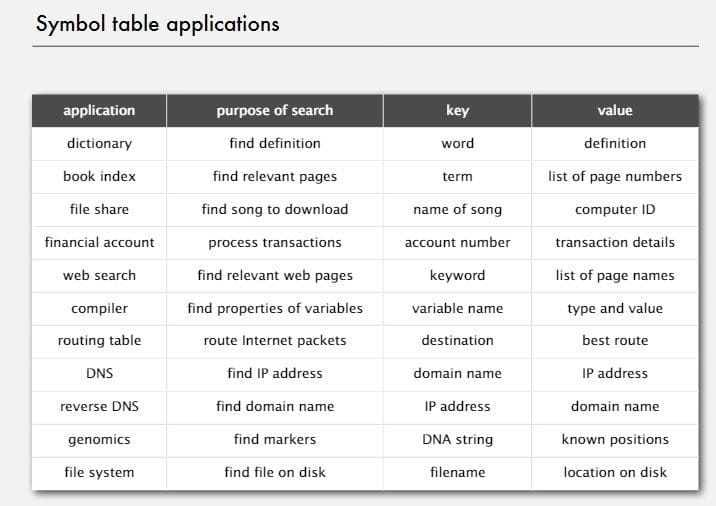# 符号表ST（1）——基本介绍

2016-05-14   |     |     |

# 1 符号表介绍和API

## 1.1 符号表介绍## 1.2 符号表API

ST() 创建一个符号表对象
void Put(Key key, Value val) 往集合中插入一条键值对记录，如果value为空，不添加
Value Get(Key key) 根据key查找value，如果没找到返回null
void Delete(Key key) 删除键为key的记录
boolean Contains(Key key) 判断集合中是否存在键为key的记录
boolean IsEmpty() 判断查找表是否为空
int Size() 返回集合中键值对的个数
Iterable Keys() 返回集合中所有的键

Ps.有一些约定要提前说一下

• 值不为null - 如果key不存在，get()返回为null
• put()会覆盖旧值

``````public boolean contains(Key key)
{  return get(key) != null;  }

public void delete(Key key)
{  put(key, null);  }
``````

# 2 键和值的约定

## 2.2 键(Key)

• Key是可比较的，即是`Comparable`的，并且使用`compareTo()`函数
• Key是泛型的
• 能用`equals()`判断相等，能用`hashCode()`获取键（这两个函数都是java的内置函数）
• 对于Key最好是使用不可变的类型(Integer,Double, String之类的)

# 3 相等性测试

`equals()`

`equals()`

• 自反性： x.equals(x) is true
• 对称性： x.equals(y) iff y.equals(x)
• 传递性： if x.equals(y) and y.equals(z), then x.equals(z)
• 不为null： x.equals(null) is false

``````public class Date implements Comparable<Date>
{
private final int month;
private final int day;
private final int year;
...
public boolean equals(Date that)
{
if (this.day   != that.day  ) return false;
if (this.month != that.month) return false;
if (this.year  != that.year ) return false;
return true;
}
}
``````

• `final`字段，不然可能会违反对称性（继承）
• 最好用`Object`,(不过这个专家们目前还在激烈争论中)
• 加入自反性，不为null的判断，并且验证类型一致性。
• 关于比较一个类中的不同变量：
• 如果比较的是一个原始类型，用==
• 如果比较的是一个对象，用equals()
• 如果比较的是一个数组，对Arrays.equals(a, b)，而不是a.equals(b)
``````public final class Date implements Comparable<Date>
{
private final int month;
private final int day;
private final int year;
...
public boolean equals(Object y)
{
if (y == this) return true;
if (y == null) return false;
if (y.getClass() != this.getClass())
return false;
Date that = (Date) y;
if (this.day   != that.day  ) return false;
if (this.month != that.month) return false;
if (this.year  != that.year ) return false;
return true;
}
}
``````